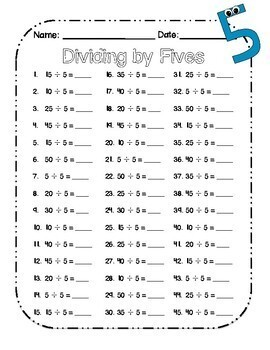DID YOU KNOW:
Seamlessly assign resources as digital activities

Learn how in 5 minutes with a tutorial resource. Try it Now

Learn More# Division Unit: Two-Week Intro to Division w/ LESSON PLANS & HOMEWORK3rd - 5th
Subjects
Standards
Resource Type
Formats Included
• PDF
Pages
54 pages

#### Also included in

1. Each unit is designed to teach the concept of multiplication/division. Building this conceptual knowledge early lets students build upon their skills.Each individual unit comes with 9 lesson plans, 9 sheets for independent practice, and 9 sheets for homework, and 1 final assessment!Altogether, you g
\$12.00
\$16.00
Save \$4.00

### Description

**A multiplication version is available here. A Combo Pack of the 2 Week Intro to Multiplication Unit AND the 2 Week Intro to Division Unit can be found at a discounted price here!**

This two-week unit is designed to introduce students to the concept of division. It does not cover all division standards. However, this is the unit I use to teach my kids the CONCEPT of division so that they have conceptual knowledge to build on throughout the year.

Included in this packet:

-9 lesson plans, complete with steps for modeling, guided practice, independent practice and homework.

-Independent practice worksheets for EVERY lesson!

-Homework sheets for EVERY lesson!

-An assessment to be given at the end of the two weeks.

If you like this unit, check out my 2-week intro unit to multiplication, which follows the same design principles to introduce the concept of multiplication:

https://www.teacherspayteachers.com/Product/Multiplication-Unit-A-Two-Week-Introduction-to-Multiplication-1982655

*****************************************************************************************

Follow my store...all new resources are 50% off during first 72 hours!

*****************************************************************************************

Total Pages
54 pages
Included
Teaching Duration
2 Weeks
Report this Resource to TpT
Reported resources will be reviewed by our team. Report this resource to let us know if this resource violates TpT’s content guidelines.

### Standards

to see state-specific standards (only available in the US).
Multiply or divide to solve word problems involving multiplicative comparison, e.g., by using drawings and equations with a symbol for the unknown number to represent the problem, distinguishing multiplicative comparison from additive comparison.
Fluently multiply and divide within 100, using strategies such as the relationship between multiplication and division (e.g., knowing that 8 × 5 = 40, one knows 40 ÷ 5 = 8) or properties of operations. By the end of Grade 3, know from memory all products of two one-digit numbers.
Understand division as an unknown-factor problem. For example, find 32 ÷ 8 by finding the number that makes 32 when multiplied by 8.
Determine the unknown whole number in a multiplication or division equation relating three whole numbers. For example, determine the unknown number that makes the equation true in each of the equations 8 × ? = 48, 5 = __ ÷ 3, 6 × 6 = ?.
Use multiplication and division within 100 to solve word problems in situations involving equal groups, arrays, and measurement quantities, e.g., by using drawings and equations with a symbol for the unknown number to represent the problem.